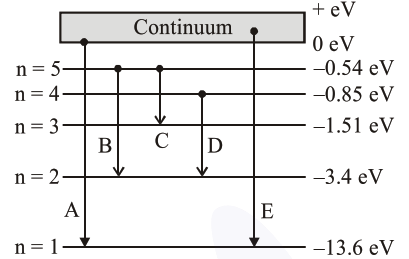# In the given figure`
Question:

In the given figure, the energy levels of hydrogen atom have been shown along with some transitions marked $A, B, C, D$ and $E$. The transitions $\mathrm{A}, \mathrm{B}$ and $\mathrm{C}$ respectively represent :1. The ionization potential of hydrogen, second member of Balmer series and third member of Paschen series.

2. The first member of the Lyman series, third member of Balmer series and second member of Paschen series.

3. The series limit of Lyman series, third member of Balmer series and second member of Paschen series.

4. The series limit of Lyman series, second member of Balmer series and second member of Paschen series.

Correct Option: , 3

Solution:

$\mathrm{A} \rightarrow$ Series limit of Lymen series.

$\mathrm{B} \rightarrow$ Third member of Balmer series.

$\mathrm{C} \rightarrow$ Second member of Paschen series.